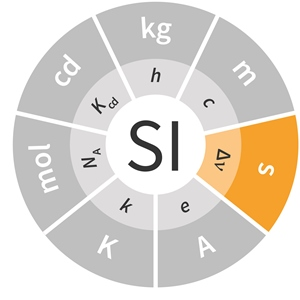# SI base unit: second (s)

The second, symbol s, is the SI unit of time. It is defined by taking the fixed numerical value of the caesium frequency ΔνCs, the unperturbed ground-state hyperfine transition frequency of the caesium-133 atom, to be 9 192 631 770 when expressed in the unit Hz, which is equal to s–1.This definition implies the exact relation ΔνCs = 9 192 631 770 Hz. Inverting this relation gives an expression for the unit second in terms of the defining constant ΔνCs:orThe effect of this definition is that the second is equal to the duration of 9 192 631 770 periods of the radiation corresponding to the transition between the two hyperfine levels of the unperturbed ground state of the 133Cs atom.